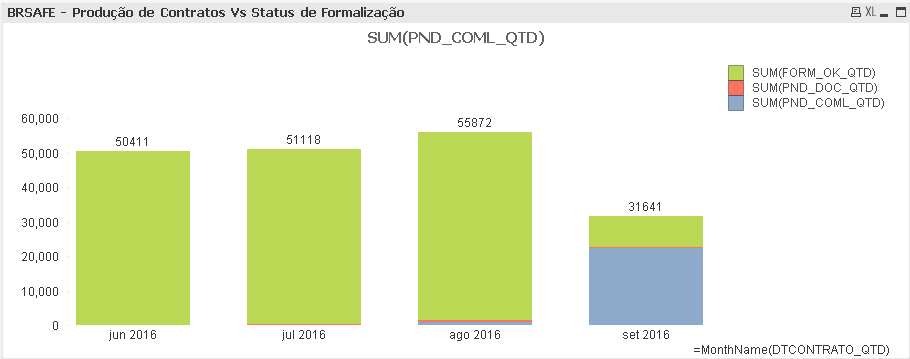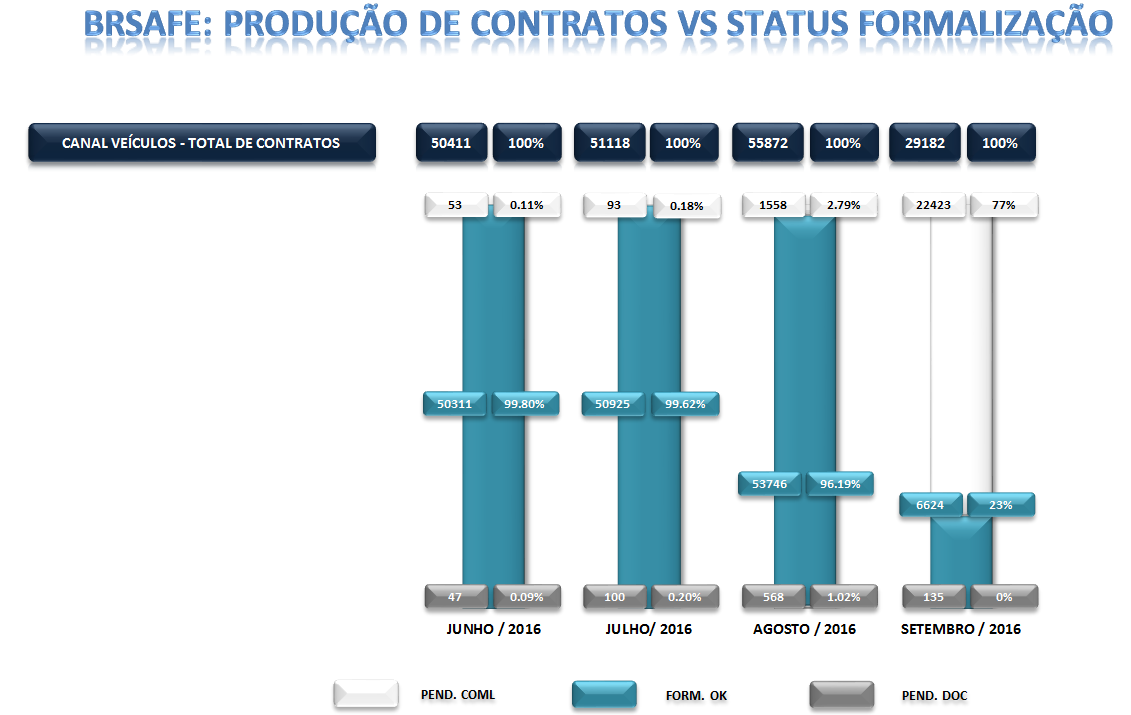# New to Qlik Sense

Discussion board where members can get started with Qlik Sense.

Announcements
Qlik® Product Spotlight: Discover what’s possible. Get more from our products.
See for yourself. Register today.
Not applicable

## Doubt about Chart 100% Stacked

Staff, good afternoon,

I am a beginner in the use of what I'm looking for help with a question on this chart.

I have the information belowand would like to know how to make the graph 100% Stacked (2):if not equal, similar to the graph (3)My Dimension:

=MonthName(DTCONTRATO_QTD)

My Expression:

SUM(PND_COML_QTD)

SUM(PND_DOC_QTD)

SUM(FORM_OK_QTD)

1 Solution

Accepted Solutions
Not applicable

## Re: Doubt about Chart 100% Stacked

Hi

Can you try this

SUM(PND_COML_QTD) / SUM(PND_COML_QTD+PND_DOC_QTD+FORM_OK_QTD)

SUM(PND_DOC_QTD) / SUM(PND_COML_QTD+PND_DOC_QTD+FORM_OK_QTD)

SUM(FORM_OK_QTD) / SUM(PND_COML_QTD+PND_DOC_QTD+FORM_OK_QTD)

Thanks,

Lakshmikandh

3 Replies
Not applicable

## Re: Doubt about Chart 100% Stacked

Hi

Can you try this

SUM(PND_COML_QTD) / SUM(PND_COML_QTD+PND_DOC_QTD+FORM_OK_QTD)

SUM(PND_DOC_QTD) / SUM(PND_COML_QTD+PND_DOC_QTD+FORM_OK_QTD)

SUM(FORM_OK_QTD) / SUM(PND_COML_QTD+PND_DOC_QTD+FORM_OK_QTD)

Thanks,

Lakshmikandh

Not applicable

## Re: Doubt about Chart 100% Stacked

Or this:

SUM(PND_COML_QTD)/RangeSum(SUM(PND_COML_QTD), SUM(PND_DOC_QTD), SUM(FORM_OK_QTD))

SUM(PND_DOC_QTD)/RangeSum(SUM(PND_COML_QTD), SUM(PND_DOC_QTD), SUM(FORM_OK_QTD))

SUM(FORM_OK_QTD)/RangeSum(SUM(PND_COML_QTD), SUM(PND_DOC_QTD), SUM(FORM_OK_QTD))

Not applicable

## Re: Doubt about Chart 100% Stacked

It worked, thanks guys

Community Browser